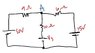# Find V3 to Make I in 10Ω Resistor Zero

• Sastronaut

## Homework Statement

In the circuit, find the value of V3 such that the current through the 10Ω resistor is zero.

V=IR

## The Attempt at a Solution

I am not sure how to apply Kirchoffs loop rule to this situation. If I want the current through the resistor to be zero then how do I apply kirchhoffs loop rule at junction A for current? I am trying to make a general statement such as I3=I1+I2...but if I3 is zero I1=I2? it makes little sense to me. any help would be greatly appreciated! thanks pf!

#### Attachments

•physics1.jpg
14.2 KB · Views: 428

## Homework Statement

In the circuit, find the value of V3 such that the current through the 10Ω resistor is zero.

V=IR

## The Attempt at a Solution

I am not sure how to apply Kirchoffs loop rule to this situation. If I want the current through the resistor to be zero then how do I apply kirchhoffs loop rule at junction A for current? I am trying to make a general statement such as I3=I1+I2...but if I3 is zero I1=I2? it makes little sense to me. any help would be greatly appreciated! thanks pf!

If the current through the 10Ω resistor is 0 you can ignore that part of the circuit (treat it as an open circuit) for the purpose of determining the current through the other resistors and the potential difference between the two marked junctions. How is the voltage provided by V3 related to the potential difference between those two junction points?

AM

I am trying to make a general statement such as I3=I1+I2...but if I3 is zero I1=I2?

Yes, that's exactly the idea.However the details of how you do that depend on how you define the directions of the two currents, I1 and I2.

So it might be something like I1 = I2, or it might be I1 + I2 = 0, all depending on how you define their directions.

it makes little sense to me.

Give it a try, and when you're finished, plug the numbers back in and you'll see (assuming no mistakes were made) that the current through the 10 Ohm resistor is 0, and the potential at point A relative to the bottom node is V3.

[Edit: Or just follow Andrew Mason's advice. I just noticed that he posted first.]